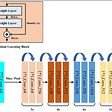# You Only Look Once: Object Detection

You only look once (YOLO) is a state-of-the-art, real-time object detection system. It uses convolutional neural network (CNN) for object detection.

Image Classification vs Object Detection

Image classification typically refers to predicting which object is present in image. So input will be set of images contain picture of animals( lets say zebra, tiger and elephant). The output will be classifying it as one of the above animal. The exact position of animal in the image does not matter.

Lets take input image of 28X28. Using RGB scale it the input will be 28X28X3 vector. This will be processes through Convolution Neural Network -combination of convolution, max pooling and fully connected layer. Final output will be 3X1 vector ( it will probability for for each class of animal). Applying SoftMax function on this vector will give exact predicted classification for image. For further details on CNN check the below link.

Object detection on the other hand predicts the location of object along with the object type. Using the same example as above, the CNN will give a vector that will have

1. If any of objects we are interested in is there or not i.e. 0,1.
2. Location of object using coordinate of center and height and width of box surrounding the object.
3. c1,c2,c3 are to depict which object it is ( we are looking for tiger , elephant and zebra)

Below is the parameter vector. This will be explained Output Vector section.

YOLO: Background

YOLO was outcome of paper published in 2015 — You Only Look Once: Unified, Real-Time Object Detection.

The official implementation is done by DarkNet and is available on github.

Overview

YOLO divides image into regions. It then predicts the possibility of object present in that region. It also predicts the bounding boxes for the object detected. It then eliminates multiple overlapping boxes- to make sure one object is surrounded in one box only.

YOLO: Steps

1. Takes the input image. Assume input image is 256X256. So RGB scale it will be represented as vector 256X256X3
2. This input image is passed through CNN.
3. To understand how final prediction works — lets understand the what the output we are looking for .

Output Vector:

The image will be divided in regions. In this example we will assume the output is divide 9 region (i.e. 3 X 3). Assuming that we want to detect ship and birds- 2 class of objects in images

So for each of 9 cell on the image grid above we need following as output

Below image shows the bounding box and associated information

In Grid G, since there is no image , associated metrics will be

In Grid D, the image below explain the numbers

h and b are normalized by taking them as fraction of height and breadth of complete image. So if h=.15 and b is .3 in above image after normalizing it

h(normalized) = .15/3=.05 and b= .3/3=.1 ( Total dimeson of image is 3,3)

The height and weight of box may be grater what grid to which they below, but at its normalized using the breadth and width of image — its always less than 1.

In the case above the dimension of output will be 3X3X7.

What we have seen till now is grid that captures image of one class. But that's not always true. For example in in Grid E. Its has both ship and bird. To handle such “Anchor Box” are used.

Anchor Box

Lets say we want to predict 2 objects per grid. In such case the dimension of out vector will change to 2X7 =14. The Metrix will be like

Any number of anchor box can be defined per grid but typically its not more than 5. In normal case we divide the output image in 19X19 grid, so 5 anchor box are sufficient.

Summary

1. We have input image lets say 265X256. Input vector to CNN is 256X256X3
2. This is fed to CNN.
3. Assumption: we want out image to be 3X3 grid ( normally for good prediction its 19X19) , max 2 objects per grid( i.e. 2 anchor box) and 3 types of objects to be identified
4. The CNN layer should produce output vector of dimension 3X3X(2X(1+4+3))= 3X3X(2X8)=3X3X16

5. Intersection over Union(IoU) and Non-Max Suppression: Before making final perdition, we can eliminate some bounding box predicted in output layer

IoU:

It uses area predicted box of object and actual box of object to eliminate predictions. If intersection areas of predicted box and actual box is i , then

IoU= i/(Sum of area of predicted + actual box)

If this IoU < some threshold we can eliminate that box. This threshold is typically .5 or above.

Non-Max Suppression

We eliminate the box that have probability less than certain threshold.

For box that remains , we pick box with highest probability and eliminate all box that have high IoU with this highest probability box.

Same process is repeated for box with next highest probability box.

This is done till we have one box surrounding the object.

The real power of YOLO is speed at which it can detect the objects. The video below shows the power of YOLO to detect objects in real time.

For Object detection and YOLO deep Dive please go though Andrew Ng’s CNN course (Week 3- Object Detection)

https://www.coursera.org/learn/convolutional-neural-networks

--

--

--

## More from Nerd For Tech

NFT is an Educational Media House. Our mission is to bring the invaluable knowledge and experiences of experts from all over the world to the novice. To know more about us, visit https://www.nerdfortech.org/.

## UDACITY SDCE Nanodegree: Term 1- Project 1 — Finding road Lanes!## Computing the driver acceptance probability or … the DRAC of Beat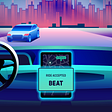## NLP News Cypher | 12.22.19## Perfect is a dirty word in Machine Learning## Taming the Edges — Part 2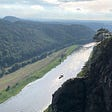## Text Similarity: Euclidian Distance VS Cosine Similarity !!!## Getting Started with Albumentation: Winning Deep Learning Image Augmentation Technique in PyTorch…## Linear Algebra with TensorFlow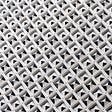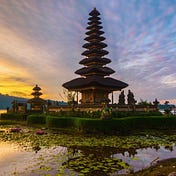## Anantech.ai

Machine Learning and System Design

## Dummies guide to Data Augmentation Using tf.Keras.## Preprocessing for Computer Vision: why your binary, one-channel, or grayscale image appear colored.## Training a Neural Network in PyTorch for a Computer Vision Task — Person Re-Identification